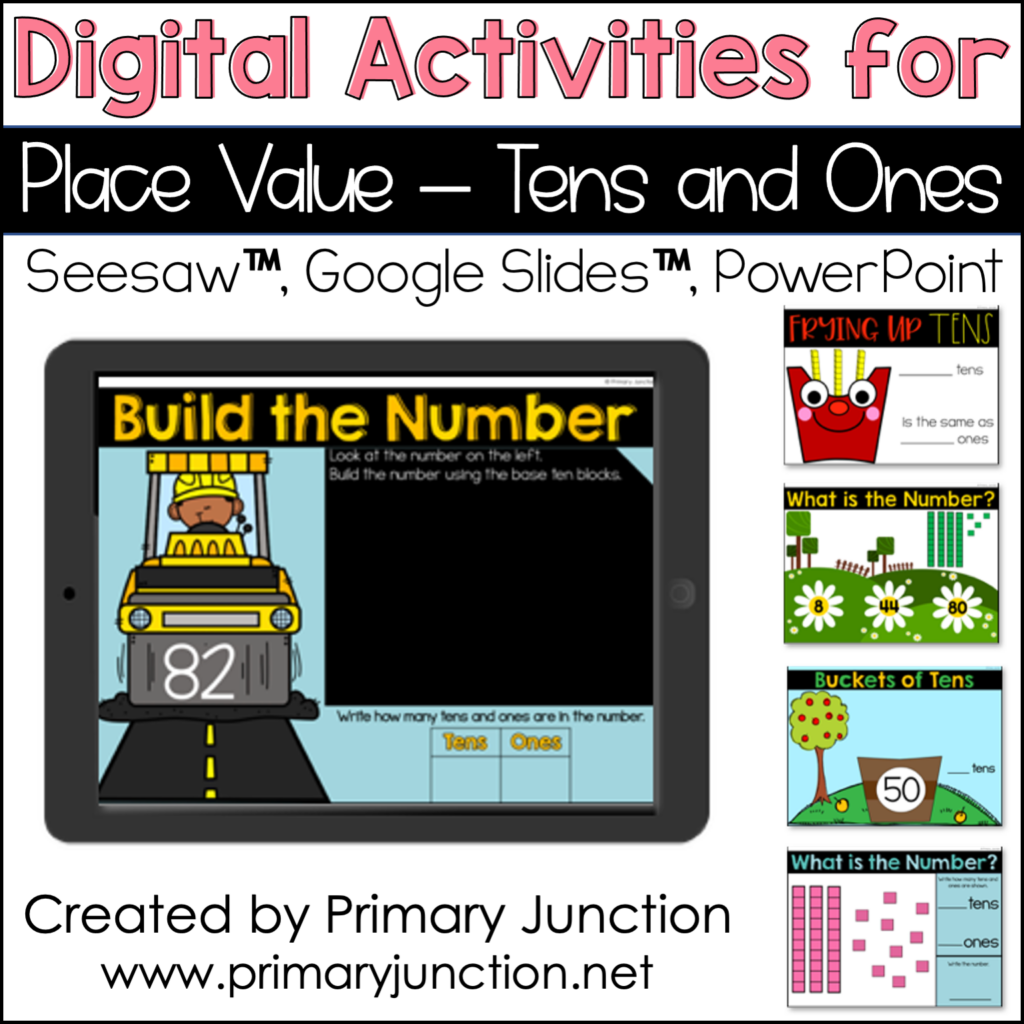# Digital Activities for Place Value Tens and Ones

\$8.00

With these activities, students have the opportunity to practice adding tens and ones to compose two-digit numbers, compare two-digit numbers using tens and ones and greater than, less than, and equal signs, identify tens in two-digit numbers, build and compose two-digit numbers using base ten blocks, show and understand the relationship between tens and ones, and identify the value of tens and ones.

These no-prep digital math activities are ready to use in PowerPoint or assign in Google Slides™️ or Seesaw™️. These digital math activities make practicing place value tens and ones skills fun and engaging for students!

All 8 activities are provided for Seesaw, Google Slides, and PowerPoint. Video directions are also included on how to access the files and assign to your students.

Categories: ,

## DescriptionMath skill practice should be fun! These 8 Place Value Tens and Ones Digital Activities provide students with the opportunity to reinforce and practice the math skills you have been working on in class!

With these activities, students have the opportunity to practice adding tens and ones to compose two-digit numbers, compare two-digit numbers using tens and ones and greater than, less than, and equal signs, identify tens in two-digit numbers, build and compose two-digit numbers using base ten blocks, show and understand the relationship between tens and ones, and identify the value of tens and ones.

These no-prep digital math activities are ready to use in PowerPoint or assign in Google Slides™️ or Seesaw™️. These digital math activities make practicing place value tens and ones skills fun and engaging for students!

All 8 activities are provided for Seesaw, Google Slides, and PowerPoint. Video directions are also included on how to access the files and assign to your students.

Easy, step-by-step written student directions are also included with each activity.

SeeSaw: The eight activities are preloaded into Seesaw. You will simply need to click to save the activities to your Seesaw library and assign them to your students.

PowerPoint: The eight files are included in this purchase. You can simply open the files to display to your students or share them through screen share.

Each activity contains 9-10 slides.

The Activities

1. Adding Tens and Ones – In this activity, students are shown a two-digit number using tens and ones. Students must write how many tens and ones are shown. Then, students must add the tens and ones together and write the number being shown with base ten blocks.

2. Comparing Numbers – In this activity, students are shown two, two-digit numbers. Students will need to write the tens and ones in each number and then compare the number using a comparison sign of less than, greater than, or equal.

3. Buckets of Tens – This activity gives students the opportunity to practice identifying tens in a number. Students are shown a two-digit number and must write the amount of tens in the given number.

4. Build the Number – In this activity, students are shown a two-digit number. They will then build the number using base ten blocks. After building the number, students will identify and write how many tens and ones compose the given number.

5. Frying Up Tens – This activity provides students with the opportunity to practice identifying tens when shown base ten blocks. Students will need to write the value/ones of the given number.

6. Groups of Ten – In this activity, students are shown groups of ten using ten frames. Students will write how many groups of ten are shown, as well as the value/ones of the number shown.

7. What is the Number? – This activity provides students with the opportunity to practice identifying a number when shown base ten blocks/tens and ones.

8. What is the Number? Tens and Ones – In this activity, students are shown base ten blocks. Students must identify how many tens and ones there are and write the value.

Please take a look at the video and PDF previews to sample this packet.

I hope you and your students enjoy using these activities!